# ❓ 你可以用纯CSS判断鼠标进入的方向吗？

## 升级版 CSS 判断鼠标进入方向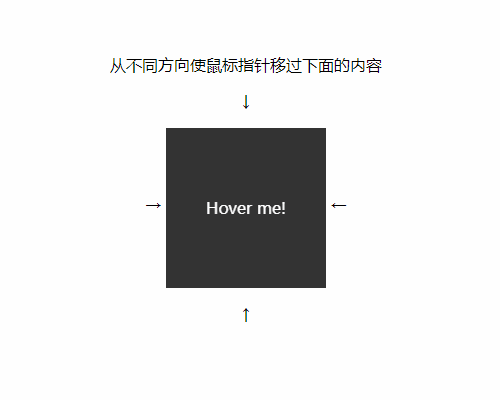CSS 判断鼠标进入方向

### 图片效果升级版 CSS 判断鼠标进入方向

## 解析

CSS 没有父选择器

HTML 如下：

``````<div class="container">
<!-- 我们需要在此处插入一些盒子，与鼠标互动，然后通过 “~ .head .eye”通用兄弟选择器，就能选中眼睛了 -->
<div class="head">
<div class="face">
<div class="mouth"></div>
<div class="eye-group">
<div class="eye eye-left"></div>
<div class="eye eye-right"></div>
</div>
</div>
</div>
</div>
``````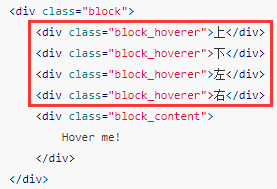CSS 判断鼠标进入方向鼠标交互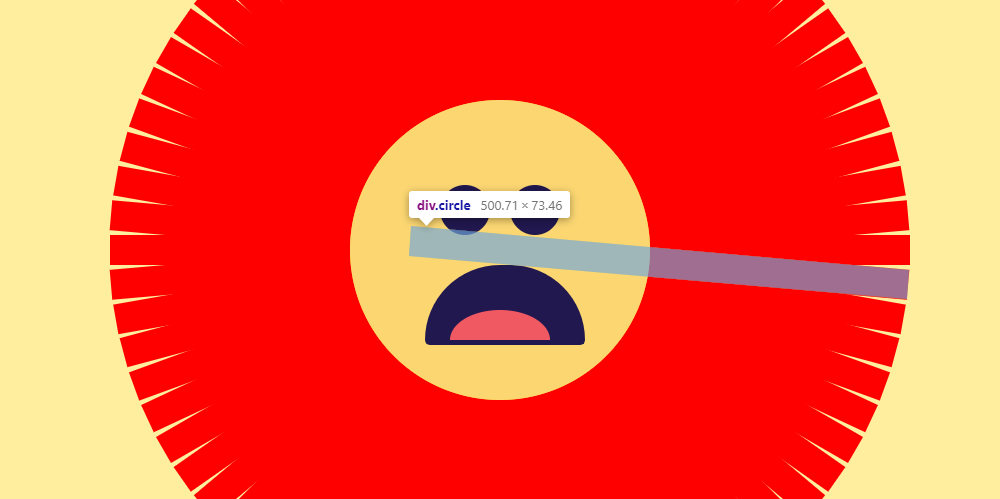升级版 CSS 判断鼠标进入方向鼠标交互

• 如何将盒子定位到圆周上
• 如何确定盒子自身旋转角度
• 如何安排盒子的宽高使得盒子完全覆盖脸的四周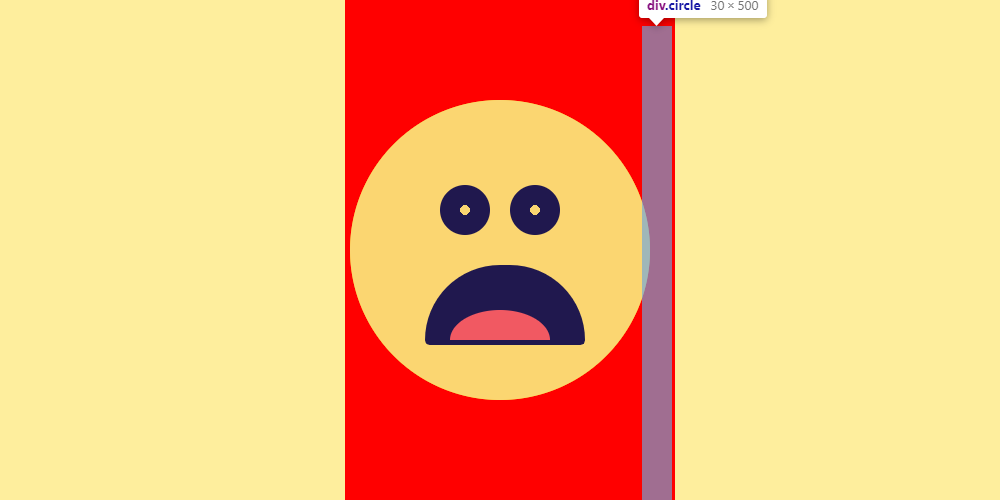盒子本身不旋转

``````// 与鼠标交互的盒子的个数
\$part: 72;
// 每个盒子间的夹角
\$part-degree: 360 / \$part;

@for \$i from 1 through \$part {
// 旋转角度这里加了 90deg 的偏移是受到了盒子的定位的影响。
// 这里可以忽略，我们只要清楚原理是根据循环确定盒子的旋转角度就好了。
transform: rotate((90 + \$i * \$part-degree) + unquote('deg'));
}
``````

CSS 中是没有正弦余弦函数的，真的么?

(→_→)

``````/** 三角函数相关 */
/** @see 代码来自 http://jimyuan.github.io/blog/2015/02/12/trigonometry-in-sass.html，感谢 */

@function fact(\$number) {
\$value: 1;
@if \$number>0 {
@for \$i from 1 through \$number {
\$value: \$value * \$i;
}
}
@return \$value;
}

@function pow(\$number, \$exp) {
\$value: 1;
@if \$exp>0 {
@for \$i from 1 through \$exp {
\$value: \$value * \$number;
}
} @else if \$exp < 0 {
@for \$i from 1 through -\$exp {
\$value: \$value / \$number;
}
}
@return \$value;
}

@function rad(\$angle) {
\$unit: unit(\$angle);
\$unitless: \$angle / (\$angle * 0 + 1);
@if \$unit==deg {
\$unitless: \$unitless / 180 * pi();
}
@return \$unitless;
}

@function pi() {
@return 3.14159265359;
}

@function sin(\$angle) {
\$sin: 0;
\$angle: rad(\$angle);
// Iterate a bunch of times.
@for \$i from 0 through 10 {
\$sin: \$sin + pow(-1, \$i) * pow(\$angle, (2 * \$i + 1)) / fact(2 * \$i + 1);
}
@return \$sin;
}

@function cos(\$angle) {
\$cos: 0;
\$angle: rad(\$angle);
// Iterate a bunch of times.
@for \$i from 0 through 10 {
\$cos: \$cos + pow(-1, \$i) * pow(\$angle, 2 * \$i) / fact(2 * \$i);
}
@return \$cos;
}
``````

``````// 脸的宽度
\$face-width: 300;
// 与鼠标交互的盒子的个数
\$part: 72;
// 每个盒子间的夹角
\$part-degree: 360 / \$part;

@for \$i from 1 through \$part {

/* 计算出盒子在圆周上的定位。需要注意的是，需要加上外围盒子的宽高及自身宽高对应的一些偏移量。 */
\$angle: (\$i / \$part) * 2 * 3.1416;
\$x: cos(\$angle) * \$face-width / 2 + 500;
\$y: sin(\$angle) * \$face-width / 2;

// 熟悉的 :nth-child 选择器。我的上一篇博客已经说到过这玩意儿了，快去看！
.box_hover:nth-child(#{\$i}) {
left: \$x + unquote('px');
top: \$y + unquote('px');
transform: rotate((90 + \$i * \$part-degree) + unquote('deg'));

// 不同的盒子的 :hover 状态，会改变其兄弟 .head 类盒子里的一些东西，这里涉及到眼睛是如何制作的，稍后会说。
&:hover ~ .head {
\$ty: sin(\$angle) * \$face-width / 50;
\$tx: cos(\$angle) * \$face-width / 50;
left: calc(50% - #{\$tx}px);
top: calc(50% - #{\$ty}px);
.eye {
&::after {
background-position: 100% 50%;
transform: rotate(
(0 + \$i * \$part-degree) + unquote('deg')
);
}
}
}
}
}
``````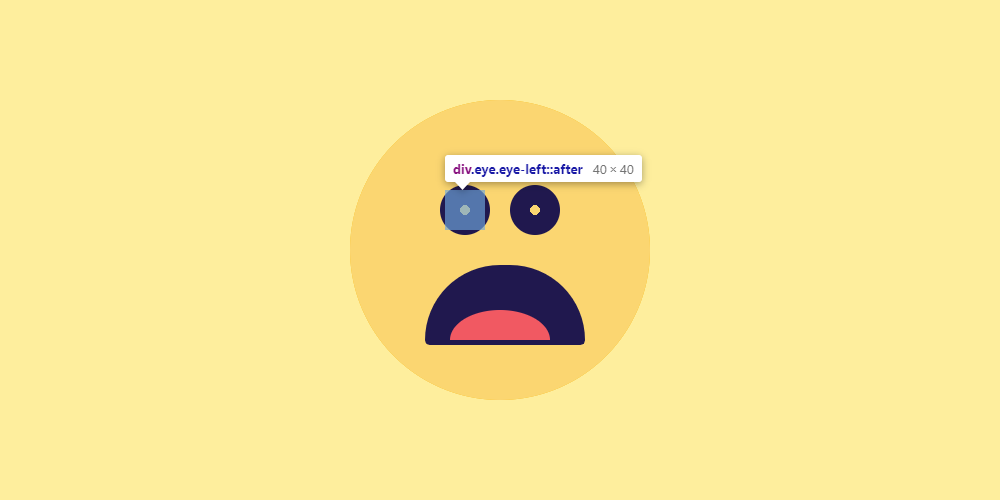画一个圆当眼睛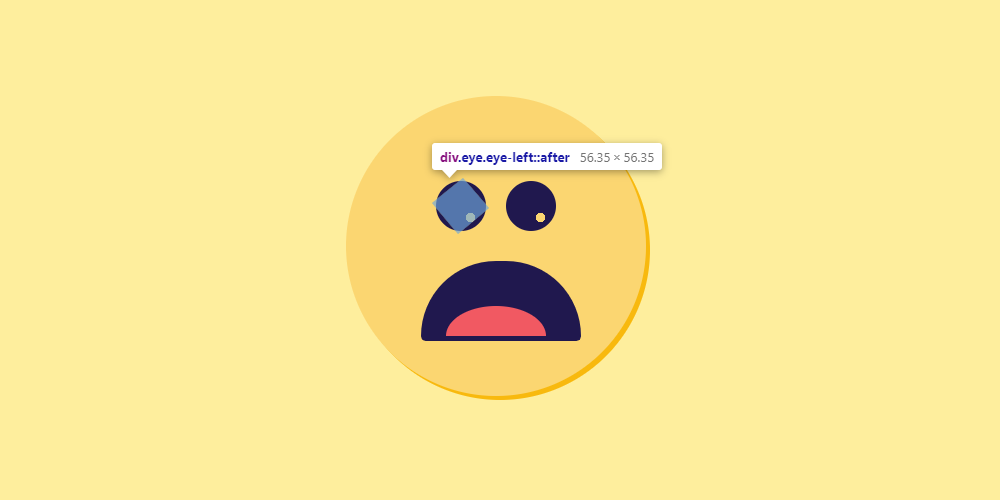改变眼睛的位置

## 源码

``````<div class="container">
<!-- 这行是 VueJS 语法，注意一下 -->
<div class="circle" v-for="item in 72"></div>
<div class="shadows"></div>
<div class="head">
<div class="face">
<div class="mouth"></div>
<div class="eye-group">
<div class="eye eye-left"></div>
<div class="eye eye-right"></div>
</div>
</div>
</div>
</div>
``````
``````/** 三角函数 @see http://jimyuan.github.io/blog/2015/02/12/trigonometry-in-sass.html */

@function fact(\$number) {
\$value: 1;
@if \$number>0 {
@for \$i from 1 through \$number {
\$value: \$value * \$i;
}
}
@return \$value;
}

@function pow(\$number, \$exp) {
\$value: 1;
@if \$exp>0 {
@for \$i from 1 through \$exp {
\$value: \$value * \$number;
}
} @else if \$exp < 0 {
@for \$i from 1 through -\$exp {
\$value: \$value / \$number;
}
}
@return \$value;
}

@function rad(\$angle) {
\$unit: unit(\$angle);
\$unitless: \$angle / (\$angle * 0 + 1);
@if \$unit==deg {
\$unitless: \$unitless / 180 * pi();
}
@return \$unitless;
}

@function pi() {
@return 3.14159265359;
}

@function sin(\$angle) {
\$sin: 0;
\$angle: rad(\$angle);
// Iterate a bunch of times.
@for \$i from 0 through 10 {
\$sin: \$sin + pow(-1, \$i) * pow(\$angle, (2 * \$i + 1)) / fact(2 * \$i + 1);
}
@return \$sin;
}

@function cos(\$angle) {
\$cos: 0;
\$angle: rad(\$angle);
// Iterate a bunch of times.
@for \$i from 0 through 10 {
\$cos: \$cos + pow(-1, \$i) * pow(\$angle, 2 * \$i) / fact(2 * \$i);
}
@return \$cos;
}

/*********************** 笑脸 */
/* 笑脸我是在 CodePen 里的某个项目基础上改的，地址忘了，汗 */

\$container-height: 500;

.container {
position: relative;
width: 1000px;
height: \$container-height + unquote('px');
overflow: hidden;
background: #feee9d;
}
.container {
* {
position: absolute;
}
*:not(.circle):before,
*:not(.circle):after {
content: '';
position: absolute;
}

\$face-width: 300;
\$circle-width: \$container-height;

/** 监听器代码 */

.circle {
position: absolute;
width: 30px;
height: \$circle-width + unquote('px');
// &:hover {
//     background: red;
// }
}
\$part: 72;
\$part-degree: 360 / \$part;
@for \$i from 1 through \$part {
\$angle: (\$i / \$part) * 2 * 3.1416;
\$x: cos(\$angle) * \$face-width / 2 - 5 + 500;
\$y: sin(\$angle) * \$face-width / 2;
.circle:nth-child(#{\$i}) {
left: \$x + unquote('px');
top: \$y + unquote('px');
transform: rotate((90 + \$i * \$part-degree) + unquote('deg'));
&:hover ~ .head {
\$ty: sin(\$angle) * \$face-width / 50;
\$tx: cos(\$angle) * \$face-width / 50;
left: calc(50% - #{\$tx}px);
top: calc(50% - #{\$ty}px);
.eye {
&::after {
background-position: 100% 50%;
transform: rotate(
(0 + \$i * \$part-degree) + unquote('deg')
);
}
}
}
}
}

/** 样式代码  */

.shadows,
.head {
border-radius: 50%;
width: \$face-width + unquote('px');
height: \$face-width + unquote('px');
transform: translate(-50%, -50%);
top: calc(50%);
left: calc(50%);
cursor: pointer;
}
.shadows {
background-color: darken(#fbd671, 20%);
}
.head {
background-color: #fbd671;
}

.face {
width: 150px;
height: 170px;
top: 75px;
left: 75px;
}

.mouth {
width: 100%;
height: 70px;
bottom: 0;
background-color: #20184e;
border: 5px solid #20184e;
border-radius: 150px 150px 10px 10px;
overflow: hidden;
&::after {
background-color: #f15962;
width: 100px;
height: 60px;
left: 20px;
top: 40px;
border-radius: 50%;
}
}

.eye-group {
top: 10px;
width: 150px;
height: 50px;
.eye {
width: 40px;
height: 40px;
background-color: #20184e;
border-radius: 50%;
border: 5px solid #20184e;
&::after {
width: 100%;
height: 100%;
top: 0;
left: 0;
background: radial-gradient(#fbd671 68%, #20184e 68%);
background-size: 10px 10px;
background-repeat: no-repeat;
background-position: 50% 50%;
transition: 0.1s;
}
&.eye-left {
left: 15px;
}
&.eye-right {
right: 15px;
}
}
}
}
``````

## 后记

### 解析

``````<div class="container">
<p class="info">盒子外部</p>
<div class="boxes">
<div class="box" v-for="item in 5">
<div class="box-inner">
<div class="left" />
<div class="right" />
<!-- .des 类是图中会变化的那些文字的容器。通过改变其伪元素的 content 改变文字内容。 -->
<p class="des"></p>
</div>
</div>
</div>
<p class="info">盒子内部</p>
</div>
``````